## Main

As graphene itself is not superconducting, phonon-mediated superconductivity must be induced by an enhancement of the electron–phonon coupling (λ),In equation (1), N(0) is the electronic density of states per spin (DOS) at the Fermi level, D is the deformation potential, and M and ωph are the effective atomic mass and phonon frequency that in metallic alloys reflect the role of the different atomic species and phonon vibrations involved in superconductivity. In undoped graphene λ is small and phonon-mediated superconductivity does not occur as the small number of carriers, intrinsic to a semimetal, leads to a vanishingly small N(0). In this respect the situation is similar to the bulk graphite case, where, without intercalation of foreign atoms superconductivity is not stabilized.

A first guess to induce superconductivity could then be to fill by rigid-band doping the carbon π-states to have enough carriers. However, besides the fact that the π-DOS grows very slowly with doping, its impact will be hindered by two major difficulties. First, even if the deformation potential related to coupling between π-bands and in-plane phonon vibrations is large and leads to Kohn anomalies13, these vibrations are highly energetic (ωph≈0.16 eV) and λ is small owing to the ωph2 factor in the denominator. Second, symmetry forbids the coupling between π-states and the softer out-of-planes vibrations.

To promote coupling to carbon out-of-plane vibrations, it is then necessary to promote new electronic states at the Fermi level as happens in GICs. Indeed, in superconducting GICs, an intercalant band (interlayer state) occurs at the Fermi level14,15 and has multiple beneficial effects on λ (ref. 20): the number of carriers is enhanced; coupling to carbon out-of-plane vibrations is promoted; and coupling to intercalant vibrations occurs with a corresponding enhancement of the deformation potential and a reduction of the effective M ωph2 term in the denominator of equation (1).

In GICs, not all types of intercalant atom leading to an interlayer state are equally effective in increasing Tc (ref. 15). The larger Tc is indeed obtained when the distance (h) between the intercalant atom and the graphite plane is smaller. The reason for this is that the closer the intercalant electrons are to the planes, the larger are the deformation potentials, D, of the intercalant and carbon out-of-plane modes15,21. This is demonstrated by the increase in Tc from BaC6 (not superconducting) to SrC6 (Tc=1.65 K; ref. 16) and finally to CaC6 (Tc=11.5 K; refs 11, 12), which correlates with the distance between the intercalant and the graphene plane (h). For the alkalis and alkaline earths considered, these distances are h(BaC6)=2.62 Å, h(SrC6)=2.47 Å and h(CaC6)=2.26 Å (ref. 15). The increase in the CaC6 critical temperature under hydrostatic pressure17 is a further confirmation of this trend.

However, a too small intercalant–graphite layer distance could also be detrimental for superconductivity. Indeed, the quantum confinement of the interlayer state in a too narrow region between the planes could result in an upshift of the intercalant band well above the Fermi energy (see Fig. 4 in ref. 14). In this case, the ionization of the intercalant atom is complete and superconductivity is totally suppressed, as in bulk LiC6 (h(LiC6)=1.85 Å).

Following these accepted guidelines for GICs, we explore the possibility to induce superconductivity in graphene by foreign-atom coverage (Fig. 1), by means of first-principles density functional theory calculations18 in the local-density approximation with the parametrization of ref. 19.

The parallel with GICs is tempting, and in particular, considering that calcium-intercalated graphite shows the highest superconducting critical temperature among GICs, the first example we consider is calcium-doped graphene and for comparison we simulated calcium-intercalated graphite. Calcium, as is the case for other alkaline metals, adsorbs in the hollow sites of graphene (Fig. 1 and Table 1).

The electronic band structure of both compounds is reported in Fig. 2. As in the bulk, the deposition of calcium on top of graphene leads to an interlayer band at the Fermi level. In passing from the bulk to the monolayer system, the removal of the quantum confinement for the electrons along the c direction lowers the energy of the interlayer state. The interlayer will then be more occupied and the carbon π-bands less doped.

However, the presence of the interlayer state at the Fermi level alone is not sufficient to guarantee large electron–phonon coupling. Indeed, a sizeable coupling between electrons and out-of-plane carbon vibrations is needed. Such coupling is due to transitions between the interlayer band and the π* states20. As the localization of the interlayer state as close as possible to the graphene layer enhances the coupling14,20,21, in Fig. 3 we compare the planar average of the interlayer charge density (calculated at the Γ-point of the Brillouin zone) in the case of bulk and monolayer CaC6. In the monolayer, the interlayer charge density spills out in the vacuum region, whereas in the bulk case, it is much more confined between the graphene and adatom layers (it has a period of 2h).

On the basis of this last consideration, we can infer that the superconducting critical temperature of the CaC6 monolayer should be lower than its bulk counterpart. To verify this hypothesis, we calculated the vibrational spectrum and the electron–phonon coupling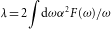of both compounds. The results are summarized in Figs 4 and 5 and Table 1. First we notice that the monolayer system is dynamically stable, not showing a tendency to displacive instabilities, with a phonon dispersion characterized by three regions: the low-energy region of adatom-related modes (up to 300 cm−1) extending up to 400 cm−1 when mixed with carbon out-of-plane modes (Cz), an intermediate region of Cz modes (400–900 cm−1) and the high-energy region characterized by C–C stretching modes.

The main difference between bulk and monolayer phonons is the softening of calcium vibrations. From inspection of the α2F(ω) (which gives the contribution of each frequency to the total electron–phonon coupling), we note the low contribution of the Cz modes (around 500 cm−1) to the total electron–phonon coupling in the monolayer case with respect to the bulk CaC6, as expected from the above considerations. Whereas the critical temperature of the bulk compound is 11.5 K (see Methods), in the monolayer case it decreases to 1.4 K.

This scenario indicates that, as a general rule, the removal of quantum confinement should be detrimental for the electron–phonon coupling when compared with the same bulk (three-dimensional periodic) system, owing to the shift in the interlayer wavefunction away from the graphene layer. Every metal-covered graphene should have (at least in the same stoichiometry) a reduced superconducting critical temperature with respect to the corresponding GIC.

However, there is at least one example among GICs (to the best of our knowledge) that should be further explored, namely the stage-1 lithium-intercalated compound, LiC6 (ref. 22). In bulk LiC6, the interlayer state is completely empty (see Fig. 2), as the strong confinement along the z direction (Fig. 3) prevents its occupation. For this reason LiC6 is not superconducting. On going from LiC6 bulk to a LiC6 monolayer, the removal of quantum confinement along the c direction (Fig. 3) could bring the interlayer to the Fermi level. This is confirmed by the calculated band structure (Fig. 2). Figure 3 shows that the spatial extension of the interlayer for lithium is the same as in calcium, but as the interlayer is strongly localized around the adatom and closer to the graphene layer, we can expect an enhancement of the total electron–phonon coupling.

The phonon spectrum confirms, even in this case, the dynamical stability of the monolayer system and the comparison with the bulk counterpart reveals that low-energy adatom modes and carbon vibrations in the direction perpendicular to the plane are strongly softened in the monolayer. This indicates that the first two phonon branches, related to the in-plane displacements of lithium atoms, and the un-dispersing Einstein mode displacing lithium and carbon atoms out-of-phase along the z direction at around 500 cm−1, should undergo enhanced electron–phonon coupling with respect to the bulk. On the contrary, in the CaC6 monolayer, adatom vibration and carbon vibrations along z are essentially at the same energy as in bulk CaC6.

We found that bulk LiC6 is a weak electron–phonon coupling λ=0.33 (0.18from carbon in-plane vibrations and 0.1 from intercalant vibrations) superconductor with an estimated superconducting critical temperature Tc=0.9 K, theoretically confirming the absence of superconductivity in LiC6 above 1 K. In the case of monolayer LiC6, the total electron–phonon coupling is λ=0.61, with a superconducting critical temperature up to 8.1 K. The main contributions to the electron–phonon coupling come from the low-energy lithium modes and carbon vibrations along z, as expected from the above considerations, with an appreciable contribution from C–C stretching modes (0.1).

Thus, we find that to induce superconductivity in graphene, it is beneficial to bring the interlayer state to the Fermi energy and to localize it as close as possible to the graphene plane. In the case of a LiC6 monolayer, the presence of the interlayer state switches on the electron–phonon coupling of Cz modes that is inactive in the bulk, and increases the contribution of intercalant modes. Graphene can be made superconducting by the deposition of lithium atoms on top of it.

As lithium readily intercalates into graphite even at 100 K (ref. 23), it is possible to incorporate lithium atoms even below the graphene sheet. This possibility, as shown by our calculations, is indeed favourable with respect to the development of a superconducting phase. In fact, the double adsorption should double the presence of the interlayer state at the Fermi level (one coming from each side of graphene) as we indeed verified.

Li2C6 shows an increased coupling in all of the frequency range owing to the additional strongly coupled interlayer bands, with an electron–phonon coupling of 1.0 giving rise to a critical temperature of around 17–18 K (see Supplementary Information).

We note again that bulk LiC6 is a candidate material to show a remarkable metal-to-superconductor transition when exfoliated to one layer. The reason is that in single-layer LiC6 the interlayer band crosses the Fermi level, enhances the electron–phonon coupling and can induce superconductivity. Our work demonstrates that superconducting properties of adatoms on graphene are quite different from their bulk GIC counterparts.

## Methods

The results reported here were obtained from first-principles density functional theory in the local density approximation19. The QUANTUM-ESPRESSO (ref. 18) package was used with norm-conserving pseudopotentials and a plane-wave cutoff energy of 65 Ry. All of the structures considered were relaxed to their minimum energy configuration following the internal forces on atoms and the stress tensor of the unit cell.

The monolayer systems were simulated in the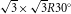in-plane unit cell (with respect to a standard graphene lattice, see Fig. 1) with one adatom per unit cell. Phonon frequencies were calculated using the linear-response technique on a phonon wave-vector mesh of 12×12 with a 14×14 uniform electron-momentum grid. The electron–phonon coupling parameter was calculated with electron momentum k-mesh up to 40×40.

The CaC6 bulk compound was simulated in the experimentally found structure with α β γ stacking11 with a uniform electron-momentum k-mesh integration of 8×8×8. The phonon frequencies were calculated on a 4×4×4 phonon-momentum mesh and the electron–phonon coupling was integrated on a 20×20×20 electron-momentum mesh.

Bulk LiC6 has an α α stacking and was simulated with an electron-momentum mesh of 12×12×10 and a 6×6×6 phonon-momentum grid for the calculation of phonon frequencies. A 30×30×25 electron-momentum mesh was used for the electron–phonon coupling.

The Eliashberg function α2F(ω) is defined as: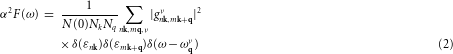where N(0) is the total density of states per spin and Nk and Nq the total numbers of k and q points, respectively. The electron eigenvalues are labelled with the band index (n and m) and the wavevector (k and k+q), while phonon frequencies with the mode number (ν) and the wavevector (q). gn k,m k+qν represents the electron–phonon matrix element. The total electron–phonon coupling λ(ω) plotted in Fig. 5 is defined as: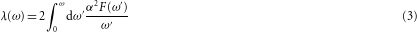The total electron–phonon coupling is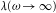. The superconducting critical temperature was estimated using the Allen–Dynes formula with μ*=0.115, which fits the experimental critical temperature measured in CaC6 GIC (ref. 11). As the doping levels considered in the present work are fairly large, the values of Tc should be weakly renormalized by the phase fluctuations of the Kosterlitz and Thouless transition24.